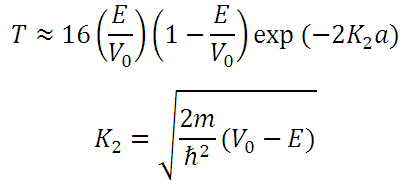# Transmission Coefficient for Tunelling of a particle through a 1-D box

This is a CalcTown calculator to calculate the transmission coefficient for tunneling of an electron through a 1-D box with a potential barrier height of Vo.

meV
meV
me
nm

#### Resultwhere

E=Total Energy of the Particle

Vo=Height of the potential Barrier

a=Length of the 1-D box

K=Second factor from the solution of the Schrodinger's wave equation in a 1-D box

me (given in the units) is the rest mass of an electron where me=9.10938291 × 10-31 kg